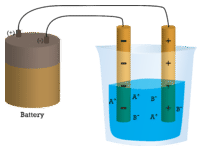# 8.9: Physical Properties of Ionic Compounds

The figure below shows just a few examples of the color and brilliance of naturally occurring ionic crystals. The regular and orderly arrangement of ions in the crystal lattice is responsible for the various shapes of these crystals, while transition metal ions give rise to the colors.Figure $$\PageIndex{1}$$: In nature, the ordered arrangement of ionic solids gives rise to beautiful crystals. (A) Amethyst - a form of quartz, $$\ce{SiO_2}$$, whose purple color comes from iron ions. (B) Cinnabar - the primary ore of mercury is mercury (II) sulfide, $$\ce{HgS}$$. (C) Azurite - a copper mineral, $$\ce{Cu_3(CO_3)_2(OH)_2}$$. (D) Vanadinite - the primary ore of vanadium, $$\ce{Pb_3(VO_4)_3Cl}$$.

## Physical Properties of Ionic Compounds

### Melting Points

Because of the many simultaneous attractions between cations and anions that occur, ionic crystal lattices are very strong. The process of melting an ionic compound requires the addition of large amounts of energy in order to break all of the ionic bonds in the crystal. For example, sodium chloride has a melting temperature of about $$800^\text{o} \text{C}$$.

### Shattering

Ionic compounds are generally hard, but brittle. Why? It takes a large amount of mechanical force, such as striking a crystal with a hammer, to force one layer of ions to shift relative to its neighbor. However, when that happens, it brings ions of the same charge next to each other (see below). The repulsive forces between like-charged ions cause the crystal to shatter. When an ionic crystal breaks, it tends to do so along smooth planes because of the regular arrangement of the ions.Figure $$\PageIndex{2}$$: (A) The sodium chloride crystal is shown in two dimensions. (B) When struck by a hammer, the negatively-charged chloride ions are forced near each other and the repulsive force causes the crystal to shatter.

### Conductivity

Another characteristic property of ionic compounds is their electrical conductivity. The figure below shows three experiments in which two electrodes that are connected to a light bulb are placed in beakers containing three different substances.Figure $$\PageIndex{3}$$: (A) Distilled water does not conduct electricity. (B) A solid ionic compound also does not conduct. (C) A water solution of an ionic compound conducts electricity well.

In the first beaker, distilled water does not conduct a current because water is a molecular compound. In the second beaker, solid sodium chloride also does not conduct a current. Despite being ionic and thus composed of charged particles, the solid crystal lattice does not allow the ions to move between the electrodes. Mobile charged particles are required for the circuit to be complete and the light bulb to light up. In the third beaker, the $$\ce{NaCl}$$ has been dissolved into the distilled water. Now the crystal lattice has been broken apart and the individual positive and negative ions can move. Cations move to one electrode, while anions move to the other, allowing electricity to flow (see figure below). Melting an ionic compound also frees the ions to conduct a current. Ionic compounds conduct an electric current when melted or dissolved in water.Figure $$\PageIndex{4}$$: In an ionic solution, the $$\ce{A^+}$$ ions migrate toward the negative electrode, while the $$\ce{B^-}$$ ions migrate toward the positive electrode.

## Summary

• Ionic compounds have high melting points.
• Ionic compounds are hard and brittle.
• Solutions of ionic compounds and melted ionic compounds conduct electricity, but solid materials do not.